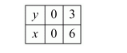# A two-digit number is 4 times the sum of its digits and twice the product of the digits.

Question:

A two-digit number is 4 times the sum of its digits and twice the product of the digits. Find the number.

Solution:

Let the digits at units and tens place of the given number be $x$ and $y$ respectively. Thus, the number is $10 y+x$.

The number is 4 times the sum of the two digits. Thus, we have

$10 y+x=4(x+y)$

$\Rightarrow 10 y+x=4 x+4 y$

$\Rightarrow 4 x+4 y-10 y-x=0$

$\Rightarrow 3 x-6 y=0$

$\Rightarrow 3(x-2 y)=0$

$\Rightarrow x-2 y=0$

$\Rightarrow x=2 y$

After interchanging the digits, the number becomes $10 x+y$.

The number is twice the product of the digits. Thus, we have $10 y+x=2 x y$

So, we have the systems of equations

$x=2 y$

$10 y+x=2 x y$

Here $x$ and $y$ are unknowns. We have to solve the above systems of equations for $x$ and $y$.

Substituting $x=2 y$ in the second equation, we get

$10 y+2 y=2 \times 2 y \times y$

$\Rightarrow 12 y=4 y^{2}$

$\Rightarrow 4 y^{2}-12 y=0$

$\Rightarrow 4 y(y-3)=0$

$\Rightarrow y(y-3)=0$

$\Rightarrow y=0$ Or $y=3$

Substituting the value of in the first equation, we haveHence, the number is $10 \times 3+6=36$.

Note that the first pair of solution does not give a two digit number.